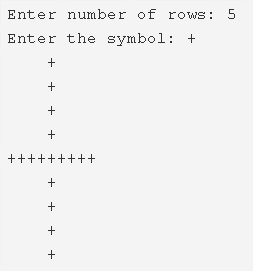# C Plus Star Pattern Program – Pattern Programs | C

C program to print a Plus Star Pattern – In this article, we will discuss the numerous methods to print a Plus Star Pattern in C programming.

Suitable examples and sample programs have also been added so that you can understand the whole thing very clearly. The compiler has also been added with which you can execute it yourself.

The methods used in this piece are as follows:

• Using For Loop
• Using While Loop
• Using Do-While Loop

It is a pretty common thing to say that Plus is one of the most used symbols of all time. It forms the base of mathematics, being one of the heavily used operators.

A plus sign is also denoted for Medical purposes. It is like the trademark symbol.As you can see in the image uploaded above, you need to enter the number of rows accordingly.

The number of rows entered for the Plus Pattern here is 5.

Then, select the symbol to print the Plus Pattern.

Funnily enough, the ‘+’ symbol is chosen to print the Plus Pattern, making it a Plus-ception.

You can see that the pattern gets printed as per the code successfully.

Thus, the methods used to do so in C programming are as follows:

## Plus Star Pattern – Using For Loop

1. Read the entered number of rows, entered characters and store the number into the variable n, character into the variable ch.

2) The 1st for loop iterates through rows with the structure for(i=1;i<=n*2-1;i++).

3) If i!=number of rows

a) The 1st inner for loop iterates through columns with the structure for(j=1;j<=n;j++).

If j==n it prints symbol.Other wise it prints space.

4) At  i=nth row

a) The 2nd inner for loop iterates through columns with the structure for(j=1;j<=n*2-1;j++).

It prints symbol.

5) The cursor comes to the next line after each row.

Output:

### Using While Loop

1. The while loop is entry checking loop. It checks the condition first for each value of i. If it is true then only it executes the code.

2) i=1, The outer while loop with the condition i<=n*2-1 iterates through rows until the condition becomes false.

3) if i!=n

a) j=1, The 1st inner while loop iterates through columns until the condition j<=n becomes false.

It prints symbol if j=n, otherwise it prints space.

j value increased by 1(go to while loop).

4) At i=nth row

a) j=1, The 2nd inner while loop iterates through columns until the condition j<=n*2-1 becomes false.

It prints symbol.j value increased by 1(go to step a).

5) Cursor comes to next line.i value increased by 1(go to outer while loop).

Output:

#### Using Do-While Loop

1. i=1, The outer do-while loop iterates through rows.

2) If i!=n

a) The 1st inner do-while loop iterates through columns.

j=1, It prints symbol if j=n, otherwise it prints space.

j value increased by 1.Checks the condition j<=n. If the condition is true then the inner loop iterates again. Repeats until the condition becomes false.

3) At i=nth row

a) j=1. the 2nd inner do-while loop iterates through columns.

Prints symbol.j value increased by 1.

Checks the condition j<=n*2-1. If the condition is true then the 2nd inner loop iterates again. Repeats until the condition becomes false.

4) Cursor comes to next line. i value increased by 1. Checks the condition i<=n*2-1. If the condition is true then the outer loop iterates again. Repeats until the condition becomes false.

x

## C Program To Check If Alphabet, Digit or Special Character | C Programs

C program to check for an alphabet, digit or special character – In this article, ...